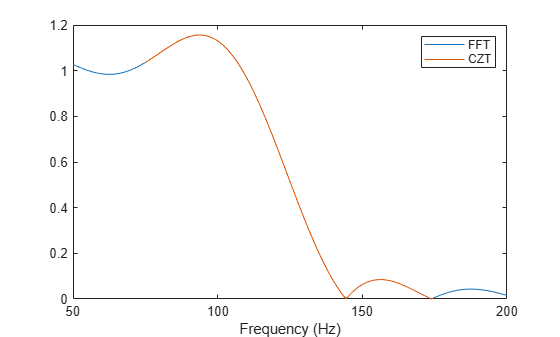# czt

Chirp Z-transform

## Syntax

```y = czt(x,m,w,a) y = czt(x) ```

## Description

`y = czt(x,m,w,a)` returns the chirp Z-transform of signal `x`. The chirp Z-transform is the Z-transform of `x` along a spiral contour defined by `w` and `a`. `m` is a scalar that specifies the length of the transform, `w` is the ratio between points along the z-plane spiral contour of interest, and scalar `a` is the complex starting point on that contour. The contour, a spiral or “chirp” in the z-plane, is given by

```z = a*(w.^-(0:m-1)) ```

`y = czt(x)` uses the following default values:

• `m` = `length(x)`

• `w` = `exp(-j*2*pi/m)`

• `a` = `1`

With these defaults, `czt` returns the Z-transform of `x` at `m` equally spaced points around the unit circle. This is equivalent to the discrete Fourier transform of `x`, or `fft(x)`. The empty matrix `[]` specifies the default value for a parameter.

If `x` is a matrix, `czt(x,m,w,a)` transforms the columns of `x`.

## Examples

collapse all

Create a random vector, `x`, of length 1013. Compute its DFT using `czt`.

```rng default x = randn(1013,1); y = czt(x);```

Use `czt` to zoom in on a narrow-band section of a filter's frequency response.

Design a 30th-order lowpass FIR filter using the window method. Specify a sample rate of 1 kHz and a cutoff frequency of 125 Hz. Use a rectangular window. Find the transfer function of the filter.

```fs = 1000; d = designfilt('lowpassfir','FilterOrder',30,'CutoffFrequency',125, ... 'DesignMethod','window','Window',@rectwin,'SampleRate',fs); h = tf(d);```

Compute the DFT and the CZT of the filter. Restrict the frequency range of the CZT to the band between 100 and 150 Hz. Generate 1024 samples in each case.

```m = 1024; y = fft(h,m); f1 = 100; f2 = 150; w = exp(-j*2*pi*(f2-f1)/(m*fs)); a = exp(j*2*pi*f1/fs); z = czt(h,m,w,a);```

Plot the transforms. Zoom in on the area of interest.

```fn = (0:m-1)'/m; fy = fs*fn; fz = (f2-f1)*fn + f1; subplot(2,1,1) plot(fy,abs(y)) axis([f1 f2 0 1.2]) title('FFT') subplot(2,1,2) plot(fz,abs(z)) axis([f1 f2 0 1.2]) title('CZT') xlabel('Frequency (Hz)')```## Diagnostics

If `m`, `w`, or `a` is not a scalar, `czt` gives the following error message:

```Inputs M, W, and A must be scalars. ```

## Algorithms

`czt` uses the next power-of-2 length FFT to perform a fast convolution when computing the z-transform on a specified chirp contour .

## References

 Rabiner, Lawrence R., and Bernard Gold. Theory and Application of Digital Signal Processing. Englewood Cliffs, NJ: Prentice-Hall, 1975, pp. 393–399.